# Length - Definition with Examples

The Complete K-5 Math Learning Program Built for Your Child

• 30 Million Kids

Loved by kids and parent worldwide

• 50,000 Schools

Trusted by teachers across schools

• Comprehensive Curriculum

Aligned to Common Core

What is Length?

Length is the term used for identifying the size of an object or distance from one point to Length is a measure of how long an object is or the distance between two points. It is used for identifying the size of an object or distance from one point to another. The length of an object is its extended dimension, that is, its longest side. For example, the length of the ruler in the picture is 15 cm.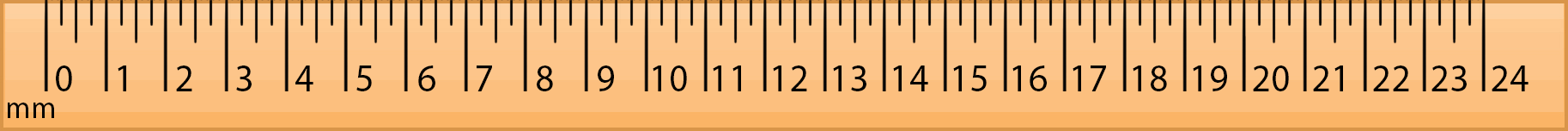Here, the arrow above the ruler denotes the length of the ruler as it is the longest side of the ruler.

Some more examples: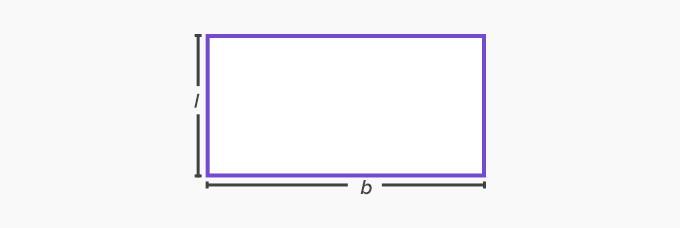The given image is of a rectangle, and for every rectangle, the length is longer than its breadth.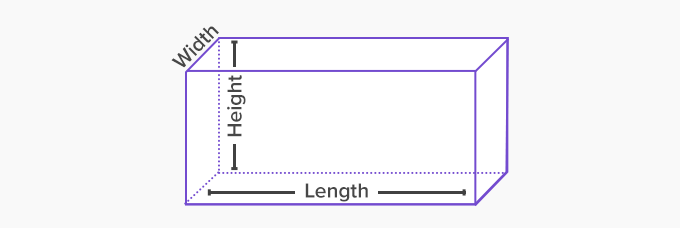Tools for measuring the length

 Vernier caliper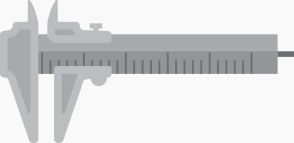TapesDifferent units of length

The standard unit of length based on the metric system is a meter (m). According to the length that needs to be measured, we can convert a meter into various units like millimeters (mm), centimeter (cm), and kilometer (km).

Centimeters and millimeters help measure smaller lengths and meters and kilometers help measure larger lengths like distance. For example, the length of the pencils can be calculated in centimeters (cm), while kilometers can measure the distance between two buildings or places.

One hundred equal divisions of a meter give a centimeter. It is written as ‘cm’. That is,

1 m = 100 cm

One thousand equal divisions of kilometer give a meter. That is,

1 km = 1,000 m

According to the length conversion charts, the different units of lengths and their equivalents are given below:

A kilometer (km), meter (m), and centimeter (cm) are the commonly used units of length.

Conversion of these units is done using the given formula.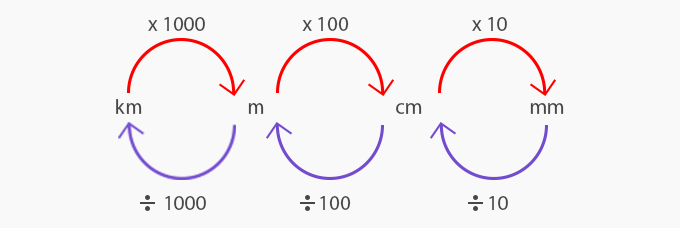Additionally, in the customary system (followed in the United States) inches, feet, yards, and miles are used as the unit for length.

The relation between the customary units is given below: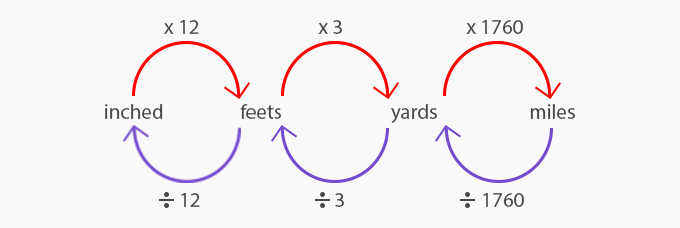Metric system and customary system

With the assortment of various units, the metric system seems quite a logical system as compared to the customary system and converting units in the metric system is much simpler than converting them in the customary system.

The United States is the last remaining nation, which is still to adopt the metric system. However, it is easy to convert units in metric to the customary system by using the given conversion.

1 meter (m) = 39.4 inches = 1.09 yards;

1 yard = 0.92 m

1 centimeter (cm) = 0.39 inches;

1 inch = 2.54 cm

 Fun Facts about lengths The word length comes from middle English ‘lengthe’ and from old English ‘lengðu’ which means — property of being long or extended in one direction. The metric system of measuring length was first adopted in France and is currently used by around 95% of the world population.

Won Numerous Awards & Honors